# NCERT Solutions for Class 12 Chemistry Chapter 2 - Solutions

Find the best NCERT Solutions at Aasoka available for Class 12 students to prepare for their board exams. Students require the finest educational resources which help them in efficiently clear their doubts related to the topics of the chapter. They can also take the help of NCERT Solutions for Class 12 to prepare revision notes which is beneficial during any exam, be it board exams or competitive exams. The content has been written by the experts as per the latest CBSE syllabus.

The chapter “Solutions” discusses the topic which includes types of solutions, ideal and non-ideal solutions, the solubility of gases and solid in a liquid, Raoult’s law, the concentration of solutions, pressure of liquid solubility, the vapour, depression of freezing point, determination of molecular masses using colligative properties, Henry’s Law, mole fraction, solid solutions, etc. These topics will help students of Class 12 to get ready for their exams in no time.
##### Question 1: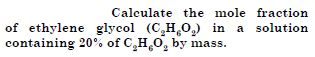20% ethylene glycol solution means that 20 g of ethylene glycol is present in 100 g of solution or 20 g of ethylene glycol is present in 80 g of water.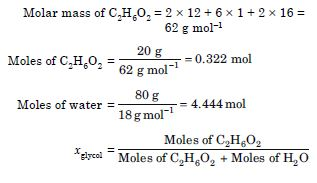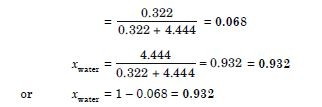##### Question 2:

Calculate the molarity of a solution containing 5 g of NaOH in 450 mL of solution.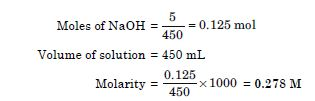##### Question 3:

Calculate the molality of 2.5 g of ethanoic acid (CH3COOH) in 75 g of benzene.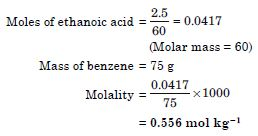##### Question 4:

If N2 gas is bubbled through water at 293 K, how many millimoles of N2 gas would be dissolved in 1 litre of water. Assume that N2 exerts a partial pressure of 0.987 bar. The KH for N2 at 293 K is 76.48 K bar.

The solubility of gas is related to its mole fraction in aqueous solution.
The mole fraction of the gas in solution.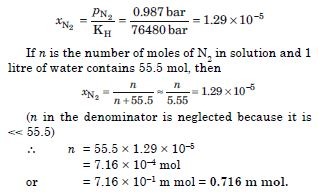##### Question 5:

Vapour pressure of chloroform (CHCl3) and dichloromethane (CH2Cl2) at 298 K are 200 mm Hg and 415 mm Hg respectively.

1. Calculate the vapour pressure of the solution prepared by mixing 25.5 g of CHCl3 and 40 g of CH2Cl2 at 298 K and
2. Mole fraction of each component in solution.

(i) Molar mass of CHCl3 = 1 × 12 + 1 × 1 + 3 × 35.5
= 119.5 g mol–1
Molar mass of CH2Cl2 = 1 × 12 + 2 × 1 + 2 × 35.5
= 85 g mol–1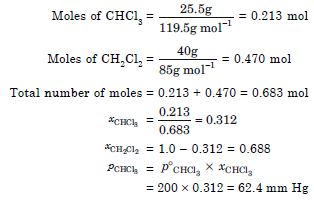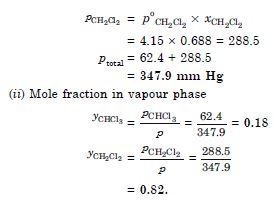##### Question 6:

The vapour pressure of pure benzene at a certain temperature is 0.850 bar. A non-volatile, non-electrolyte solid weighing 0.50 g is added to 39.0 g of benzene (molar mass 78 g mol–1). The vapour pressure of the solution, then is 0.845 bar. What is the molar mass of the solid substance?

According to Raoult’s law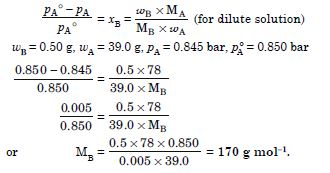##### Question 7:

18 g of glucose, C6H12O6 (molar mass = 180 g mol–1) is dissolved in 1 kg of water in a sauce pan. At what temperature will this solution boil at 1.013 bar? Kb for water is 0.52 K kg mol–1.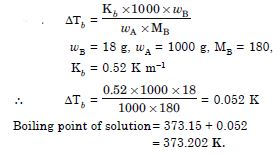##### Question 8:

The boiling point of benzene is 353.23 K. When 1.80 g of a non-volatile solute was dissolved in 90 g of benzene, the boiling point is raised to 354.11 K. Calculate the molar mass of the solute.

(Kb for benzene is 2.53 K kg mol–1)

Molar mass of a substance can be calculated as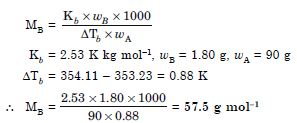##### Question 9:

45 g of ethylene glycol (C2H6O2) is mixed with 600 g of water. Calculate

1. the freezing point depression
2. the freezing point of the solution (Kf for water = 1.86 K kg ml–1)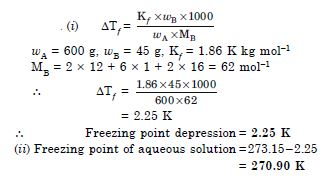##### Question 10:

1.00 g of a non-electrolyte solute dissolved in 50 g of benzene lowered the freezing point of benzene by 0.40 K. The freezing point depression constant of benzene is 5.12 K kg mol–1. Calculate the molar mass of the solute.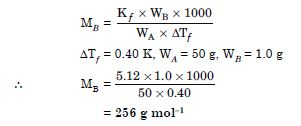##### Question 11:

200 cm3 of an aqueous solution of a protein contains 1.26 g of the protein. The osmotic pressure of such a solution at 300 K is found to be 2.57 × 10–3 bar. Calculate the molar mass of the protein. (R = 0.083 L bar mol–1)

According to Van’t Hoff equation,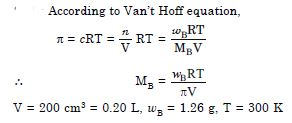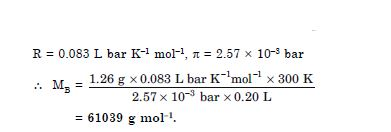##### Question 12:

2 g of benzoic acid (C6H5COOH) dissolved in 25 g of benzene shows a depression in freezing point equal to 1.62 K. Molal depression constant for benzene is 4.9 K kg mol–1. What is the percentage association of acid if it forms double molecules (dimer) in solution ?

Let us first calculate observed molar mass,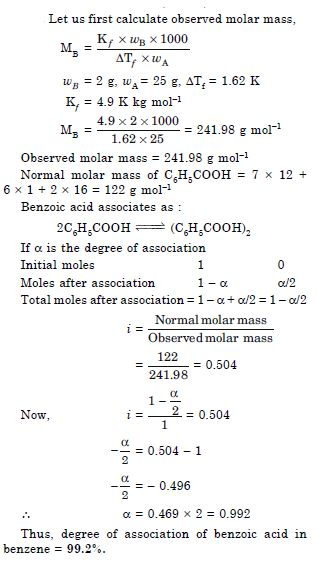##### Question 13:

0.6 mL of acetic acid
(CH3COOH) having density 1.06 g mL–1 is dissolved in 1 litre of water. The depression in freezing point observed for this strength of acid was 0.0205$°$C. Calculate the Van’t Hoff factor and dissociation constant of the acid.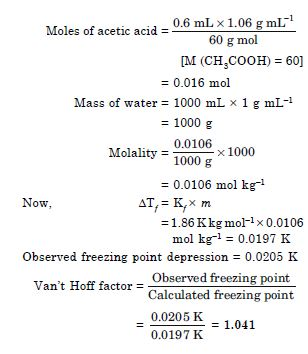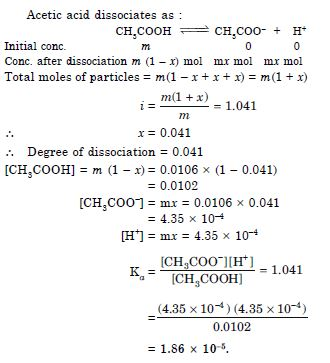##### Question 14:

Calculate the mass percentage of benzene (C6H6) and carbon tetrachloride (CCl4) if 22 g of benzene is dissolved in 122 g of carbon tetrachloride.

Total mass of solution = 22 + 122 = 144 g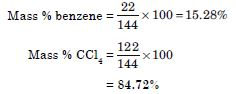##### Question 15:

Calculate the mole fraction of benzene in solution containing 30% by mass in carbon tetrachloride.

Mass of benzene = 30 g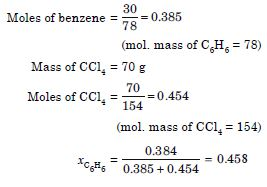##### Question 16:

Calculate the molarity of each of the following solutions :

1. 30 g of Co(NO3)2.6H2O in 4.3 L of solution.
2. 30 mL of 0.5 M H2SO4 diluted to 500 mL.

Molar mass of Co(NO3)2.6H2O
= 58.93 + 2 × 14 + 6 × 16 + 6 × 18
= 290.93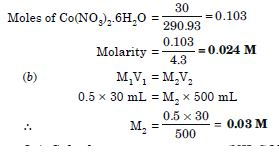##### Question 17:

Calculate the mass of urea (NH2CONH2) required in making 2.5 kg of 0.25 molal aqueous solution.

0.25 molal aqueous solution of urea means that
Moles of urea = 0.25 mol, Mass of solvent = 1000 g, Mass of urea = 0.25 × 60 = 15 g
Total mass of solution = 1000 + 15
= 1015 g
or = 1.015 kg
1. 015 kg solution contain urea 15 g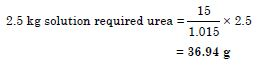##### Question 18:

Calculate (a) molality (b) molarity and (c) mole fraction of KI if density of 20% (mass/mass) aqueous solution of KI is 1.202 g mL–1.

20% solution of KI means 20 g of KI are present in 100 g of solution or 80 g of water.
Mass of KI = 20 g
Molar mass of KI = 39 + 127 = 166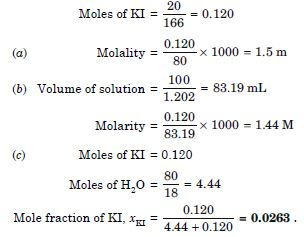##### Question 19:

H2S, a toxic gas with rotten egg like smell is used for qualitative analysis. If the solubility of H2S in water at STP is 0.195 m. Calculate Henry’s law constant.

0.195 m solution means that 0.195 moles of H2S is dissolved in 1 kg of water.

Moles of H2S = 0.195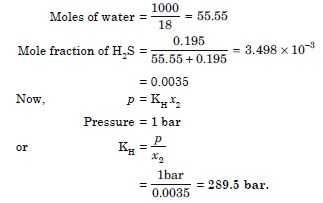##### Question 20:

Henry’s law constant for CO2 in water is 1.67 × 108 Pa at 298 K. Calculate the quantity of CO2 in 500 mL of soda water when packed under 2.5 atm CO2 pressure at 298 K.

KH = 1.67 × 108 Pa
p = 2.5 atm = 2.5 × 101.325 × 103 Pa
= 2.533 × 105 Pa
Now, p = KH x2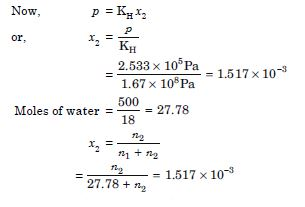n2 = 0.0419 + 0.0015 n2
0.9985 n2 = 0.0419 or n2 = 0.0420
Amount of CO2 dissolved = 0.0420 × 44 = 1.85 g.

##### Question 21:

The vapour pressure of pure liquids A and B are 450 and 700 mm Hg at 350 K respectively. Find out the composition of the liquid mixture if total pressure is 600 mm Hg. Also find the composition of vapour phase.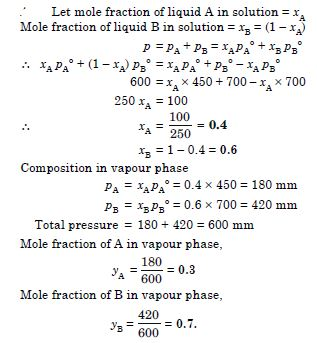##### Question 22:

Vapour pressure of pure water at 298 K is 23.8 mm Hg. 50 g of urea (NH2CONH2) is dissolved in 850 g of water. Calculate the vapour pressure of water for this solution and its relative lowering.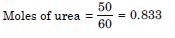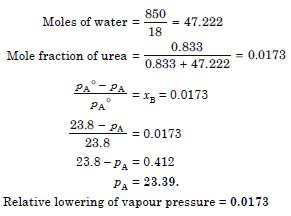##### Question 23:

Boiling point of water at 750 mm Hg is 99.63$°$C. How much sugar is to be added to 500g of water such that it boils at 100$°$C.

$∆$Tb = 100 – 99.63 = 0.37$°$

##### Question 24:

Calculate the mass of ascorbic acid (vitamin C, C6H8O6) to be dissolved in 75 g of acetic acid to lower its melting point by 1.5$°$C. Kf = 3.9 K kg mol–1.

$∆$Tb = 1.5$°$C
M = 6 × 12 + 1 × 8 + 6 × 16 = 176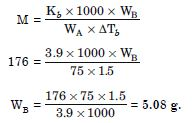##### Question 25:

Calculate the osmotic pressure in Pascals exerted by a solution prepared by dissolving 1.0 g of polymer of molar mass 185,000 in 450 mL of water at 37$°$C.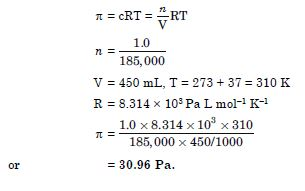##### Question 26:

Define the term solution. How many types of solutions are formed? Write briefly about each type with an example.

A solution is a homogeneous mixture of two or more substances whose composition can be varied.

On the basis of physical state of the components of solution, there are the following nine types of solutions: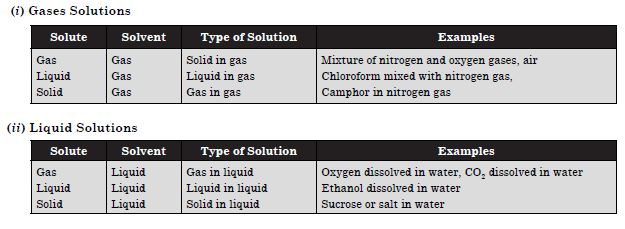##### Question 27:

Give an example of a solid solution in which the solute is a ga

##### Question 28:

Define the following terms : (i) Mole fraction (ii) Molality (iii) Molarity (iv) Mass percentage.

1. (i) Mole fraction. It is the ratio of the number of moles of one component to the total number of moles of all the components present in the solution. For example, if a binary solution has nA moles of one component and nB moles of second component, then mole fraction of component A(xA) and B(xB) is :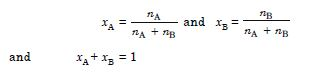2. Molality. It is the number of moles of solute dissolved per 1000 g (or 1 kg) of solvent. If n2 moles of solute are dissolved in w1 g of solvent , then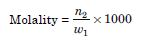3. Molarity. It is the number of moles of solute dissolved per litre of the solution. If n2 moles of solute are dissolved in V mL of the solution, then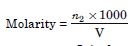4. Mass percentage. It is the amount of solute (in grams) per 100 g of the solution. If w2 g of solute are dissolved in w1 g of solvent, then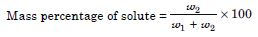##### Question 29:

Concentrated nitric acid used in the laboratory work is 68% nitric acid by mass in aqueous solution. What should be molarity of such sample of the acid if the density of solution is 1.504 g mL–1?

68% HNO3 means that 68 g HNO3 is present in 100 g of solution.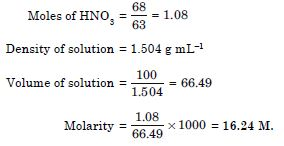##### Question 30:

A solution of glucose in water is labelled as 10% w/w, what would be the molality and mole fraction of each component in the solution ? If the density of solution is 1.2 g mL–1, then what shall be the molarity of the solution?

10 % (w/w) solution of glucose means that 10 g of glucose is present in 100 g of solution or in 90 g of water.

1. Calculation of molality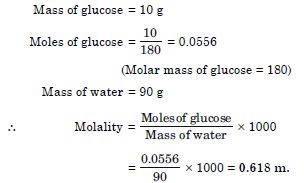2. Calculation of molarity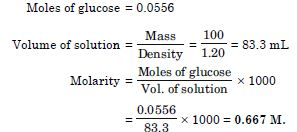3. Calculation of mole fraction of components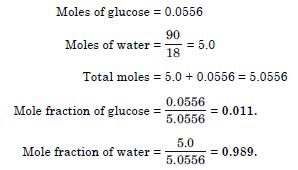##### Question 31:

How many mL of 0.1 M HCl are required to react completely with 1 g mixture of Na2CO3 and NaHCO3 containing equimolar amounts of both?

Let the amount of Na2CO3 be x in the mixture.
Amount of NaHCO3 = 1 – x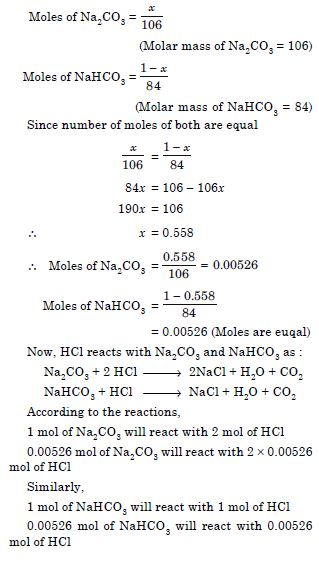Total moles of HCl required to react with mixture of NaHCO3 and Na2CO3
= 2 × 0.00526 + 0.00526
= 0.01578 mol
To calculate volume of 0.1 M HCl corresponding to 0.01578 mol, we have
0.1 mol of 0.1 M HCl is present in 1000 mL
0.01578 mol of 0.1 M HCl is present in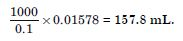##### Question 32:

A solution is obtained by mixing 300 g of 25% solution and 400 g of 40% solution by mass. Calculate the mass percentage of the resulting solution.

300 g of 25% solution contains solute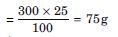400 g of 40% solution contains solute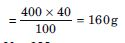Total solute = 75 + 160 = 235 g
Total solution = 300 + 400 = 700 g
% of solute in the initial solution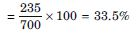% of water in the final solution = 100 – 33.5 = 65.5%.

##### Question 33:

An antifreeze solution is prepared from 222.6 g of ethylene glycol (C2H6O2) and 200 g of water. Calculate the molality of the solution. If the density of the solution is 1.072 g mL–1, then what shall be the molarity of the solution ?

1. Calculation of molality Mass of ethylene glycol = 222.6 g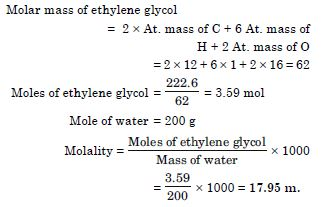2. Calculation of molarity Moles of ethylene glycol = 3.59 mol Mass of solution = 200 + 222.6 = 422.6 g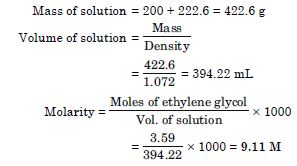##### Question 34:

A sample of drinking water was found to be severely contaminated with chloroform (CHCl3). Supposed to be a carcinogen. The level of contamination was 15 ppm (by mass) : (i) express this in percent by mass, (ii) determine the molality of chloroform in the water sample.

15 ppm (by mass) means 15 g of CHCl3 is present in 106 g of solution.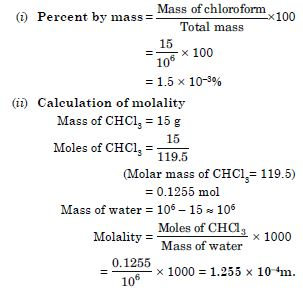##### Question 35:

What role does the molecular interactions play in solution of alcohol and water?

There are strong hydrogen bonding in alcohol molecules as well as water molecules. On mixing alcohol and water, the molecular interactions become weak. Therefore, they show positive deviation from ideal behaviour. As a result, the solution will have higher vapour pressure and lower boiling point than that of alcohol and water.

##### Question 36:

Why do gases always tend to be less soluble in liquids as the temperature is raised?

The dissolution of a gas in a liquid is exothermic process. Therefore, in accordance with Le Chatelier’s principle, with increase in temperature, the equilibrium shifts in the backward direction.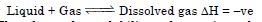Therefore, the solubility of gas in solution decreases with rise in temperature.

##### Question 37:

State Henry’s law and mention some important applications ?

Henry’s law states that at a constant temperature, the solubility of a gas in a liquid is directly proportional to the pressure of the gas. If mole fraction (x) is the measure of the solubility of the gas, then
x $\mathrm{\alpha }$ p
or x = k′p

##### Question 38:

The partial pressure of ethane over a solution containing 6.56 × 10–3 g of ethane is 1 bar. If the solution contains 5.00 × 10–2 g of ethane, then what shall be the partial pressure of the gas?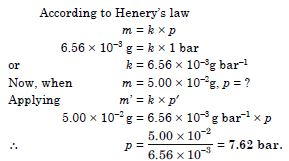##### Question 39:

What is meant by positive and negative deviations from Raoult’s law and how is the sign of ${∆}_{\mathrm{mix}}$ related to positive and negative deviations from Raoult’s law?

Positive deviation : When the total vapour pressure of the solution is greater than the corresponding vapour pressure exerted on the basis of Raoult’s law, the solution is said to show positive deviation.

and ptotal = pA + pB is always greater than (p0A xA + p0B xB)

Let us consider a solution of ethyl alcohol and cyclohexane. In alcohol, the molecules are held together by hydrogen bonding as shown below :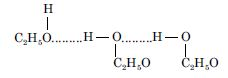When cyclohexane is added to ethyl alcohol, the molecules of cyclohexane tend to occupy the spaces between alcohol molecules. Consequently, some hydrogen bonds in alcohol molecules break and the attractive forces in alcohol molecules are weakened. As a result there is slight increase in vapour pressure upon mixing.

Negative deviation : When the total vapour pressure of the solution is less than the corresponding vapour pressure exerted on the basis of Raoult’s law, the solution is said to show negative deviation.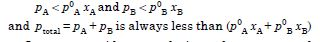Let us consider a solution of acetone and chloroform. When acetone and chloroform are mixed, there are new attractive forces due to intermolecular hydrogen bonding. Thus, the attractive forces become stronger and the escaping tendency of each liquid from the solution decreases. As a result, the vapour pressure of the solution is slightly less than that is expected for an ideal solution.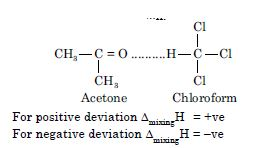For positive deviation ${∆}_{\mathrm{mixing}}$H = +ve
For negative deviation ${∆}_{\mathrm{mixing}}$H = –ve

##### Question 40:

An aqueous solution of 2% non-volatile solute exerts a pressure of 1.004 bar at the normal boiling point of the solvent. What is the molar mass of the solute ?

Vapour pressure of pure water at the boiling point = 1 atm = 1.013 bar
Vapour pressure of solution = 1.004 bar
Mass of solute = 2 g
Mass of solution = 100 g
Mass of solvent = 100 – 2 = 98 g
Applying Raoult’s law,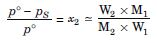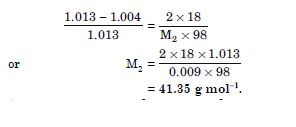##### Question 41:

Heptane and octane form ideal solution. At 373 K, the vapour pressures of the two liquid components are 105.2 kPa and 46.8 kPa respectively. What will be the vapour pressure of a mixture of 26.0 g of heptane and 35 g of octane ?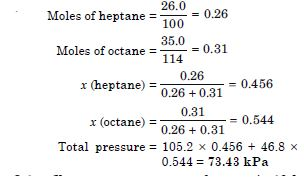##### Question 42:

The vapour pressure of water is 12.3 kPa at 300K. Calculate vapour pressure of 1 molal solution of a non-volatile solute in it.

Mole fraction of solute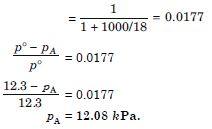##### Question 43:

Calculate the mass of a non-volatile solute (molar mass 40 g mol–1) which should be dissolved in 114 g octane to reduce its vapour pressure to 80%.

The vapour pressure is reduced to 80% on the addition of a non-volatile solute so that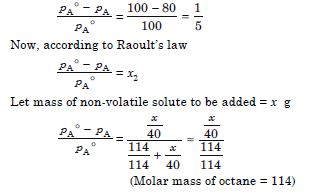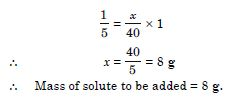##### Question 44:

A solution containing 30 g of non-volatile solute exactly in 90 g of water has a vapour pressure of 2.8 kPa at 298 K. Further, 18 g of water is then added to the solution, the new vapour pressure becomes 2.9 kPa at 298K. Calculate (i) molar mass of the solute,
(ii) vapour pressure of water at 298 K.

wB = 30 g, wA = 90 g, pA = 2.8 kPa
According to Raoult’s law,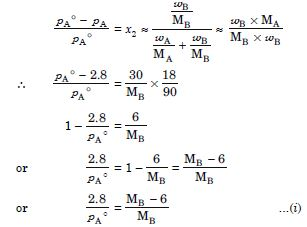Similarly,
wB = 30 g, wA = 90 + 18 = 108 g, pA = 2.9 kPa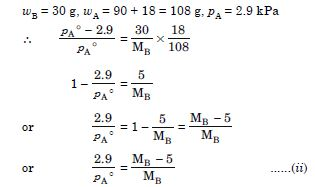Dividing Eqn. (i) by (ii)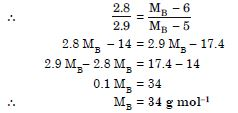Substituting the value of MB in Eq. (i)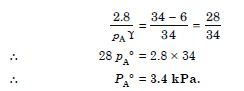##### Question 45:

A 5% solution (by mass) of cane sugar in water has freezing point of 271 K. Calculate the freezing point of 5% glucose in water if freezing point of pure water is 273.15 K.

For cane sugar,For glucose solution,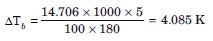##### Question 46:

Two elements A and B form compounds having formula AB2 and AB4. When dissolved in 20 g of benzene (C6H6), 1 g of AB2 lowers the freezing point by 2.3 K, whereas 1.0 g of AB4 lowers it by 1.3 K. The molar depression constant for benzene is 5.1 K kg mol–1. Calculate atomic masses of A and B.

Let us first calculate molar masses of AB2 and AB4. For AB2 compoundFor AB4 compound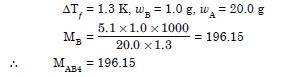Let a is the atomic mass of A and b is the atomic mass of B, then
MAB2 = a + 2b = 110.87 ...(i)
MAB4 = a + 4b = 196.15 ...(ii)
Subtracting Eqn. (ii) from (i)
– 2b = – 85.28
$\therefore$ b = 42.64
Substituting the value of b in Eqn (i)
a + 2 × 42.64 = 110.87
$\therefore$ a = 110.87 – 85.28 = 25.59
Atomic mass of A = 25.59 and Atomic mass of
B = 42.64.

##### Question 47:

At 300 K, 36 g of glucose present per litre in its solution has an osmotic pressure of 4.98 bar. If the osmotic pressure of the solution is 1.52 bar at the same temperature. What would be its concentration ?

According to Van’t Hoff equation.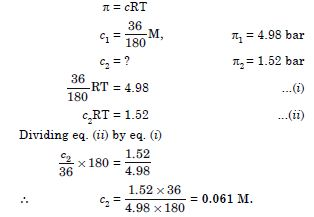##### Question 48:

Suggest the most important type of intermolecular attractive interaction in the following pairs. (i) n-hexane and n-octane (ii) I2 and CCl4 (iii) NaClO4 and water (iv) methanol and acetone (v) acetonitrile (CH3CN) and acetone (C3H6O).

van der Waal’s interactions, (ii) van der Waal’s interactions, (iii) ion-dipole, (iv) hydrogen bonding (v) dipole-dipole interactions.

##### Question 49:

Based on solute-solvent interactions, arrange the following in order of increasing solubility in n-octane and explain. Cyclohexane, KCl, CH3OH, CH3CN.

KCl, CH3OH, CH3CN, Cyclohexane.

##### Question 50:

Amongst the following compounds, identify which are insoluble, partially soluble and highly soluble in water ? (i) phenol, (ii) toluene, (iii) formic acid, (iv) ethylene glycol, (v) chloroform, (vi) pentanol.

(i) partially soluble (ii) insoluble (iii) highly soluble (iv) highly soluble (v) insoluble (vi) partially soluble.

##### Question 51:

If the density of some lake water is 1.25 g mL–1 and contains 92 g of Na+ ions per kg of water. Calculate the molarity of Na+ ions in the lake.

Mass of Na+ ions = 92 g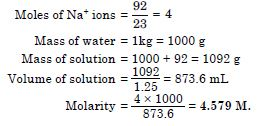##### Question 52:

If the solubility product of CuS is 6 × 10–6. Calculate the maximum molarity of CuS in aqueous solution.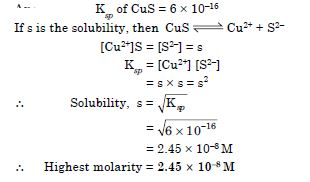##### Question 53:

Calculate the mass percentage of aspirin (C9H8O4) in acetonitrile (CH3CN) when 6.5 g of C9H8O4 is dissolved in 450 g of CH3CN.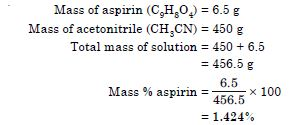##### Question 54:

Nalorphene (C19H21NO3), similar to morphine, is used to combat withdrawal symptoms in narcotic users. Dose of nalorphene generally given is 1.5 mg. Calculate the mass of 1.5 × 10–3 m aqueous solution required for the above dose.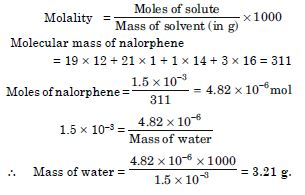##### Question 55:

Calculate the amount of benzoic acid (C6H5COOH) required for preparing 250 mL of 0.15 M solution in methanol.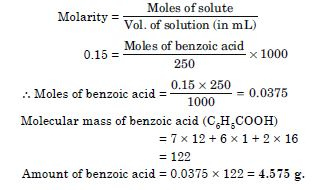##### Question 56:

The depression in the freezing point of water observed for the same amount of acetic acid, trichloroacetic acid and trifluoroacetic acid increases in the order given above. Explain briefly.

The acids can be arranged in the following order of depression in freezing point : acetic acid < trichloroacetic acid < trifluroacetic acid
This can be explained on the basis of degree of ionization which depends upon the strength of the acid. Trifluroacetic acid is more acidic than trichloroacetic acid which is more acidic than acetic acid. Therefore, degree of ionization of these will decreases as : trifluoroacetic acid > trichloroacetic acid > acetic acid Greater the degree of ionization, greater will be ‘i’ and hence depression in freezing point.

##### Question 57:

Calculate the depression in the freezing point of water when 10 g of CH3CH2CHClCOOH is added to 250 g of water. Ka = 1.4 × 10–3,
Kf = 1.86 K kg mol–1.

Molar mass of CH3CH2CHClCOOH
= 4 (12) + 7 (1) + 2 (16) + 1 (35.5)
= 122.5 g mol–1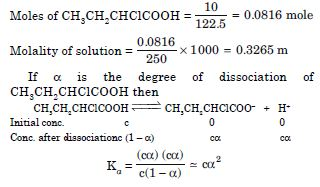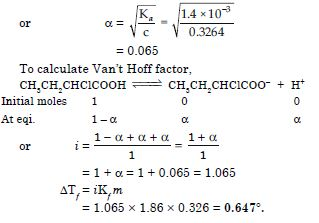##### Question 58:

19.5 g of CH2FCOOH is dissolved in 500 g of water. The depression in the freezing point of water observed is 1.0$°$C. Calculate the Van’t Hoff factor and dissociation constant of fluoroacetic acid.

Molecular mass of CH2FCOOH
= 2 × 12 + 3 × 1 + 1 × 19 + 2 × 16 = 78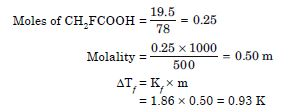Van’t Hoff factor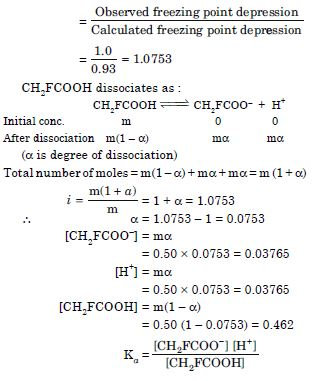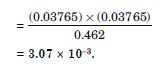##### Question 59:

Vapour pressure of water at 293 K is 17.535 mm Hg. Calculate the vapour pressure of water at 293 K when 25 g of glucose is dissolved in 450 g of water.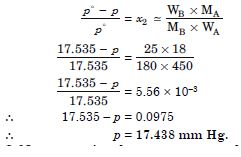##### Question 60:

Henry’s law constant for the molality of methane in benzene at 298 K is 4.27 × 105 mm Hg. Calculate the solubility of methane in benzene at 298 K under 760 mm Hg.

p = k × C
760 mm = 4.25 × 105 mm × C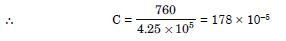or = 1.78 × 10–3.

##### Question 61:

100 g of liquid A (molar mass 140 g mol–1) was dissolved in 1000 g of liquid B (molar mass 180 g mol–1). The vapour pressure of pure liquid B was found to be 500 torr. Calculate the vapour pressure of pure liquid A and its vapour pressure in the solution if the total vapour pressure of the solution is 475 torr.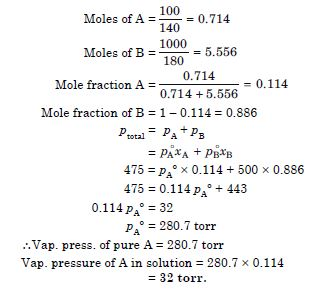##### Question 62:

Vapour pressure of pure acetone and chloroform at 328 K are 741.8 mm Hg and 632.8 mm Hg respectively. Assuming that they form ideal solution over the entire range of composition, plot ptotal, pchloroform and pacetone as a function of xacetone. The experimental data observed for different compositions of mixture is :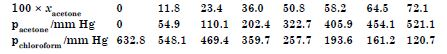Plot this data also on the same graph paper. Indicate whether it has positive deviation or negative deviation from the ideal solution.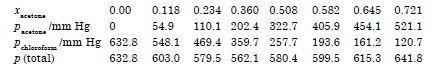The plot of p(total) dips downwards and therefore, the solution shows negative deviation from ideal behaviour.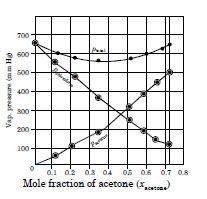##### Question 63:

Benzene and toluene form ideal solution over the entire range of composition. The vapour pressure of pure benzene and toluene at 300 K are 50.71 mm Hg and 32.06 mm Hg respectively. Calculate the mole fraction of benzene in vapour phase if 80 g of benzene is mixed with 100 g of toluene.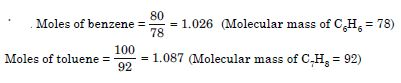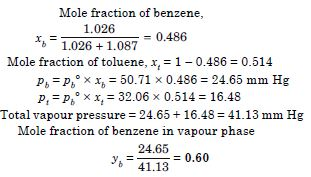##### Question 64:

The air is a mixture of number of gases. The major components are oxygen and nitrogen with approximate proportion of 20% is to 79% by volume at 298 K. The water is in equilibrium with air at a pressure of 10 atm. Henry’s law constants for oxygen and nitrogen at 298 K are 3.30 × 107 mm and 6.51 × 107 mm respectively. Calculate the composition of these gases in water.

Total vapour pressure of air over water = 10 atm. The partial pressure of N2 and O2 are :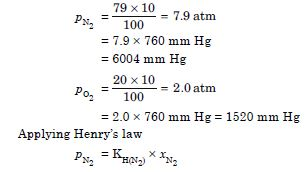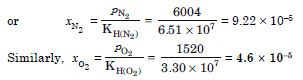##### Question 65:

Determine the amount of CaCl2
(i = 2.47) dissolved in 2.5 litre of water such that its osmotic pressure is 0.75 atm at 27$°$C.

or CaCl2,
i = 2.47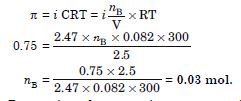##### Question 66:

Determine the osmotic pressure of a solution prepared by dissolving 25 mg of K2SO4 in 2 litre of water at 25$°$ C, assuming that it is completely dissociated.

If K2SO4 is completely dissociated,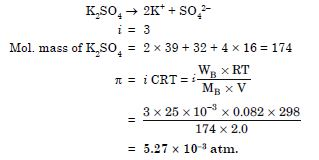##### Question 67:

Components of a binary mixture of two liquids A and B were being separated by distillation. After some time separation of components stopped and composition of vapour phase became same as that of liquid phase. Both the components started coming in the distillate. Explain why this happened

Since both the components are coming in the distillate and composition of liquid and vapour phase become same, this shows that liquids have formed azeotropic mixture. Therefore, these components cannot be separated at this stage by distillation.

##### Question 68:

Explain why on addition of 1 mol of NaCl to 1 litre of water, the boiling point of water increases, while addition of 1 mol of methyl alcohol to one litre of water decreases its boiling point.

NaCl is a non volatile solute, therefore, addition of NaCl to water lowers the vapour pressure of water. As a result, boiling point of water increases. On the other hand, methyl alcohol is more volatile than water, therefore its addition increases the total vapour pressure over the solution. As a result, boiling point of water decreases.

##### Question 69:

Explain the solubility rule “like dissolves like” in terms of intermolecular forces that exist in solutions.

A substance dissolves in a solvent if the intermolecular interactions are similar in both the components. For example, polar solutes dissolve in polar solvents and non polar solutes in non polar solvents. Thus, we can say “like dissolves like”.

##### Question 70:

Concentration terms such as mass percentage, ppm, mole fraction and molality are independent of temperature, however molarity is a function of temperature. Explain.

Molarity of a solution is defined as the number of moles of solute dissolved per litre of solution. Since volume depends on temperature and changes with change in temperature, therefore, the molarity will also change with change in temperature. On the other hand, mass does not change with change in tempera-ture, and therefore, concentration terms such as mass percentage, mole fraction and molality which do not involve volume are independent of temperature.

##### Question 71:

What is the significance of Henry’s Law constant KH?

Higher the value of Henry’s law constant KH, the lower is the solubility of the gas in the liquid.

##### Question 72:

Why are aquatic species more comfortable in cold water in comparison to warm water?

At a given pressure, the solubility of oxygen in water increases with decrease in temperature. Therefore, the concentration of oxygen in sea is more in cold water and thus presence of more oxygen at lower temperature makes the aquatic species more comfortable in cold water.

##### Question 73:
1. Explain the following phenomena with the help of Henry’s law.
1. Painful condition known as bends.
2. Feeling of weakness and discomfort in breathing at high altitude.
2. Why soda water bottle kept at room temperature fizzes on opening ?

(a) (i) Deep sea divers depends upon compressed air for breathing at high pressure under water. The compressed air contains N2 in addition to O2, which are not very soluble in blood at normal pressure. However, at great depths when the diver breathes in compressed air from the supply tank, more N2 dissolves in the blood and other body fluids because the pressure at that depth is far greater than the surface atmospheric pressure. When the diver comes towards the surface, the pressure decreases, N2 comes out of the body quickly forming bubbles in the blood stream. These bubbles restrict blood flow, affect the transmission of nerve impulses. The bubbles can even burst the capillaries or block them and starve the tissues of O2. This condition is called “the bends,” which are painful and dangerous to life.

(ii) At high altitudes, the partial pressure of O2 is less than that at the ground level. This results in low concentration of oxygen in the blood and tissues of the people living at high altitudes or climbers. The low blood oxygen causes climbers to become weak and unable to think clearly known as anoxia.

(b) To increase the solubility of CO2 in soft drinks, the soda water bottles are sealed under high pressure. When the bottle is opened at room temperature under normal atmosphere conditions, the pressure inside the bottle decreases to atmospheric pressure and excess CO2 fizzes out.

##### Question 74:

Why is the vapour pressure of an aqueous solution of glucose lower than that of water?

In pure liquid water, the entire surface of liquid is occupied by the molecules of water. When a non volatile solute, such as glucose is dissolved in water some of the surface is covered by non volatile glucose molecules. Therefore, the fraction of surface covered by the solvent molecules decreases. As a result, number of solvent molecules escaping from the surface also gets reduced and consequently the vapour pressure of aqueous solution of glucose is reduced.

##### Question 75:

How does sprinkling of salt help in clearing the snow covered roads in hilly areas? Explain the phenomenon involved in the process.

When salt is spread over snow covered roads, it lowers the freezing point of water to such an extent that water does not freeze to form ice. As a result, the snow starts melting from the surface and therefore, it helps in clearing the roads. Hence, common salt acts as de-icing agent.

##### Question 76:

What is “semi permeable membrane”?